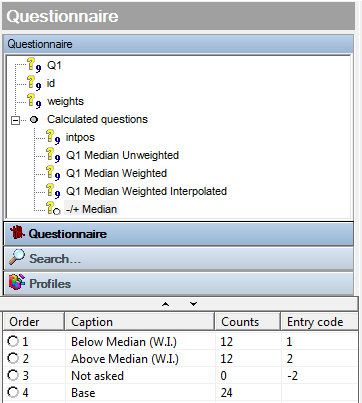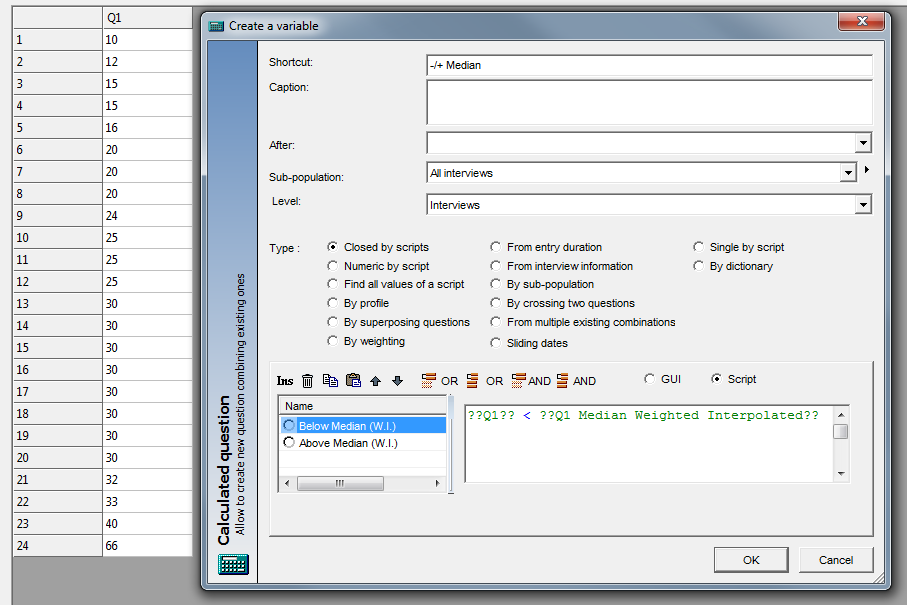Scroll

# Dynamic Median Split Variable

Follow
 Summary This article is the first in a series of articles that will go through examples of using aggregate script syntax new to Askia 5.3.5.0. Specifically, this article demonstrates how to calculate a weighted median of a set of respondents, store it in a variable and then use it to create other variables for tabulation. Applies to Askia Analyse. Written for Researchers, Data Processors, Analysts. Keywords Aggregated script, Median, Interpolation, Weighting, Dynamic, Split.

The .qes file and portfolio containing the example discussed is attached: Aggregated Scripts Examples 1.rar

An easy way to think about ‘Aggregated Scripts’ is that, amongst other things, they give you access to aggregated calculations (e.g. counts, mean, median, standard deviation, min, max, sum) for a set of respondents.  How could we use this in a real life situation?

Imagine the requirement is for us to tabulate a split of respondents who rated less than the Median at Q1 vs respondents who rated more than the Median at Q1 and we want this to be automatically updated when new data is added.

First let’s see how we would work out the Median and some different ways to do this: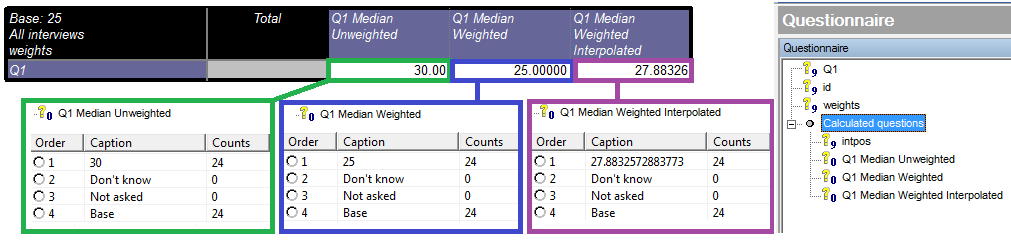The syntax for the unweighted median is as follows:  Q1.data.median(0)

The data object accesses the data for the question and then we get the Median by using ‘.median(0)’ – the (0) part is the instruction not to use interpolation whereas (1) would use interpolation.

Interpolation:

We have the 24 data points in the example .qes lined up from smallest to largest. The value in the middle is our median but here we have no middle value because we have a 12th value (25) and a 13th value (30). Without interpolation it takes the first value above the given split point (30). However, with interpolation, it works out the mean of the two middle values: (27.5).

For weighted data it’s a weighted average calculation: see full explanation.

The syntax for the weighted median is as follows: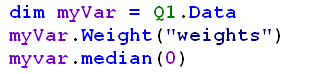The syntax for the weighted, interpolated median is as follows: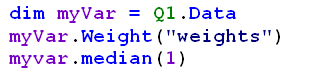If I write these examples into a ‘Numeric by script’ calculation, I am in a position to set up my final split variable as the following: